# High School Math : Finding Derivative at a Point

## Example Questions

### Example Question #1 : Finding Derivative At A Point

Find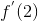if the functionis given by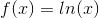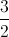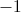Explanation:

To find the derivative at, we first take the derivative of. By the derivative rule for logarithms,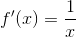Plugging in, we get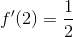### Example Question #2 : Finding Derivative At A Point

Find the derivative of the following function at the point.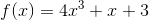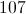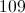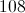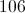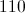Explanation:

Use the power rule on each term of the polynomial to get the derivative,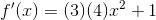Now we plug in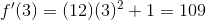### Example Question #11 : Derivatives

Let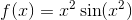. What is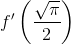?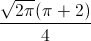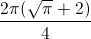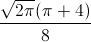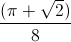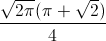Explanation:

We need to find the first derivative of f(x). This will require us to apply both the Product and Chain Rules. When we apply the Product Rule, we obtain: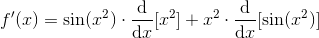In order to find the derivative of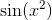, we will need to employ the Chain Rule.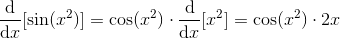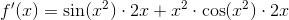We can factor out a 2x to make this a little nicer to look at.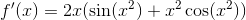Now we must evaluate the derivative when x =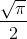.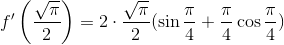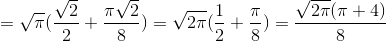The answer is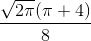.